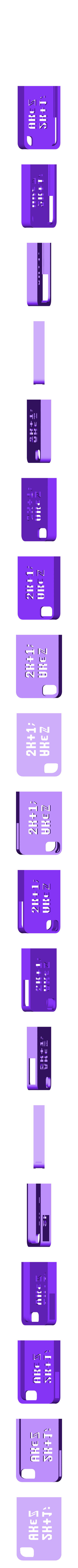Skip to content

# Odd Case## 3D model description

Want a case for your iPhone that is the epitome of odd? Now you can print your own case that is the true definition of odd! (At least in the mathematical sense)

In the field of mathematics an odd integer is defined as being equal to 2k+1; kâˆˆâ„¤. In words that the rest of us can understand, an integer is odd if it is equal to some integer multiplied by 2 and had an 1 added to it. The "kâˆˆâ„¤" part means that "k" is an element(âˆˆ) of the set of all integers(â„¤). The formula on this printed case is the set of all odd integers (e.g. -3, -1, 1, 3), this is denoted by the upside down A (âˆ€) meaning that for all k where k is an integer, the number that is equal to 2k+1 is odd.

On a side note, the development of this case can be written as a nice set of implication statements:
When you take discrete math, you become confused. When you become confused, you are not sure of the actual definition of things. When you are not sure of the actual definition of things, you start making strange puns. When you start making strange puns, you design iPhone cases like this. Therefore, unless you want to start making strange puns, don't take discrete math.

In the end, if you are still reading this, just think, you will have the oddest iPhone case around! So odd, you may not even know what it means.

## 3D printing settings

1) Download and print one copy of top.stl and bottom.stl
2) Remove current case from iPhone
3) Add your new case to your iPhone
4) Explain to your friends the strange markings on your case and hope that they don't think you are crazy.

• 3D model format: STL